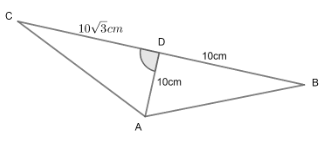Courses
Courses for Kids
Free study material
Free LIVE classes
MoreLIVE
Join Vedantu’s FREE Mastercalss

# The altitude AD of $\Delta ABC$ in which $\angle A$ is obtuse and AD = 10cm. If BD = 10 cm and $CD=10\sqrt{3}cm$, determine $\angle A$.Verified
359.4k+ views
Hint: Calculate the value of $\angle CAD\ and\ \angle BAD$using trigonometric ratios like sine, cosine and tangent.In $\Delta ACD$, since $\Delta ACD$ is a right angled triangle,
\begin{align} & \tan \left( \angle CAD \right)=\dfrac{CD}{AD} \\ & =\dfrac{10\sqrt{3}}{10} \\ & =\sqrt{3} \\ & \Rightarrow \angle CAD={{\tan }^{-1}}\sqrt{3} \\ & \Rightarrow \angle CAD=\dfrac{\pi }{3} \\ \end{align}
We will now find $\angle BAD$, since $\Delta ABD$ is a right angled triangle,
\begin{align} & \tan \left( \angle BAD \right)=\dfrac{BD}{AD} \\ & =\dfrac{10}{10} \\ & =1 \\ & \Rightarrow \angle BAD={{\tan }^{-1}}1 \\ & \Rightarrow \angle BAD=\dfrac{\pi }{4} \\ & \angle A=\angle CAD+\angle BAD \\ & =\dfrac{\pi }{3}+\dfrac{\pi }{4} \\ & =\dfrac{7\pi }{12} \\ & =105{}^\circ \\ \end{align}
Hence $\angle A$, as asked to us in the question = $\dfrac{7\pi }{12}\ or\ 105{}^\circ$.

Note: The question can also be solved using the cosine and sine formula after finding the length of hypotenuse of each triangle using Pythagoras Theorem.

Last updated date: 23rd Sep 2023
Total views: 359.4k
Views today: 5.59k